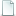## Think Bayes - Bayesian Statistics Made Simple Allen B. Downeythinkbayes.pdf 2.37MB
Type: Paper
Tags: paper, bayes, book

Bibtex:
```@article{,
title= {Think Bayes - Bayesian Statistics Made Simple},
journal= {},
author= {Allen B. Downey},
year= {},
url= {},
abstract= {Think Bayes is an introduction to Bayesian statistics using computational methods.

The premise of this book, and the other books in the Think X series, is that if you know how to program, you can use that skill to learn other topics.

Most books on Bayesian statistics use mathematical notation and present ideas in terms of mathematical concepts like calculus. This book uses Python code instead of math, and discrete approximations instead of continuous mathematics. As a result, what would be an integral in a math book becomes a summation, and most operations on probability distributions are simple loops.

I think this presentation is easier to understand, at least for people with programming skills. It is also more general, because when we make modeling decisions, we can choose the most appropriate model without worrying too much about whether the model lends itself to conventional analysis. Also, it provides a smooth development path from simple examples to real-world problems.

Think Bayes is a Free Book. It is available under the Creative Commons Attribution-NonCommercial 3.0 Unported License, which means that you are free to copy, distribute, and modify it, as long as you attribute the work and don't use it for commercial purposes.},
keywords= {paper, bayes, book},
terms= {},